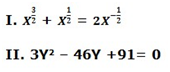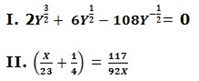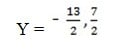# RRB PO Prelims Quantitative Aptitude (Day-04)

Dear Aspirants, Our IBPS Guide team is providing new series of Quantitative Aptitude Questions for RRB PO 2020 Prelims so the aspirants can practice it on a daily basis. These questions are framed by our skilled experts after understanding your needs thoroughly. Aspirants can practice these new series questions daily to familiarize with the exact exam pattern and make your preparation effective.

Start Quiz

Direction (1-5): What should come in the place of question mark in the following number series?

1) 3, 10, 32, 111, 460, ?

A) 2215

B) 2320

C) 2310

D) 2314

E) 2315

2) 40, 48, 60, 78,  ? , 147.42

A) 105.5

B) 106.5

C) 105.3

D) 106.3

E) 112.3

3) 16, 19, 24, 33, 50, ?

A) 83

B) 99

C) 66

D) 77

E) 102

4) 58, 128, 260, 442, 714, ?

A) 1129

B) 1135

C) 1056

D) 1133

E) 1115

5)  999, 1647, 2457, 3429, 4563, ?

A) 5868

B) 5879

C) 5869

D) 5881

E) 5859

Direction (6-10): Following question contains two equations as I and II. You have to solve both equations and determine the relationship between them

6)A) X > Y

B) X ≤ Y

C) Relationship between X and Y can’t be established

D) X ≥ Y

E) X < Y

7)

I) 7X2– 35√3X+126 = 0

II) 9Y– 27√3Y-270= 0

A) X > Y

B) X ≤ Y

C) Relationship between X and Y can’t be established

D) X ≥ Y

E) X < Y

8)A) X > Y

B) X ≤ Y

C) Relationship between X and Y can’t be established

D) X ≥ Y

E) X < Y

9)

I) 55X– 32X – 63 = 0

II) 33Y2– 49Y + 18 = 0

A) X > Y

B) X ≤ Y

C) Relationship between X and Y can’t be established

D) X ≥ Y

E) X < Y

10)

I) 2x– 25x+ 75 =0

II) 4y+ 12y = 91

A) X > Y

B) X ≤ Y

C) Relationship between X and Y can’t be established

D) X ≥ Y

E) X < Y

Directions (1-5) :

3 x 1 + (1 x 7) = 10

10 x 2 + (2 x 6) = 32

32 x 3 + (3 x 5) = 111

111 x 4 + (4 x 4) = 460

460 x 5 + (5 x 3) = 2315

40 x 1.2 = 48

48 x 1.25 = 60

60 x 1.3 = 78

78 x 1.35 = 105.3

105.3 x 1.4 = 147.42

16 + (21 + 1) = 19

19 + (22 + 1) = 24

24 + (23 + 1) = 33

33 + (24 + 1) = 50

50 + (25 + 1) = 83

58 + 7 x 10 = 128

128 + 11 x 12 = 260

260 + 13 x 14 = 442

442 + 17 x 16= 714

714 + 19 x 18 = 1056

12– 93 = 999

153 – 123 = 1647

18– 15= 2457

21– 183 = 3429

24– 213 = 4563

27– 243 = 5859

Directions (6-10) :Multiply both the sides with

X2+X = 2

X2+X– 2 = 0

X (X +2) – 1(X+2) = 0

(X-1) (X+2) = 0

X = +1, -2

II. 3Y2– 46Y +91= 0

3Y2 – 39Y -7Y + 91= 0

3Y (Y – 13) – 7 (Y – 13) = 0

(3Y -7) (Y-13) = 0

Y = 7/3 , 13

Hence, X < Y.

I) 7X2– 35√3X+126= 0

X2 – 53X+18 = 0

X2 – 23X-33X+18 = 0

(X- 23) (X – 33) = 0

X = 23, 33

II) 9Y– 27√3Y-270= 0

Y– 33Y-30 = 0

Y– 53Y+23Y-30 = 0

(Y+23) (Y – 53) = 0

Y = -23, +53

Hence, no relation between X and Y.2Y2 + 6Y – 108 = 0

Y2 + 3Y – 54 = 0

Y2 + 9Y –  6Y- 54 = 0

Y (Y+9) – 6 (Y+9) = 0

(Y + 9) (Y – 6) = 0

Y = +6, -94X2+ 23X – 117 = 0

4X2+ 36X – 13X -117 = 0

4X(X+9) – 13(X+9) = 0

(X+9) (4X -13) = 0

X = -9, 13/4

Hence, No relation between X and Y.

I) 55X– 32X – 63 = 0

55X– 77X +45X – 63 = 0

11X (5X – 7) + 9 (5X -7) = 0

(5X -7) (11X +9) = 0

X= 7/5, -9/11

II) 33Y2– 49Y + 18 = 0

33Y2 – 22Y -27Y + 18 = 0

11Y (3Y -2) – 9(3Y-2) = 0

(3Y-2) (11Y-9) = 0

Y = 2/3, 9/11

Hence, No relation between X and Y.

I) 2x– 25x + 75=0

2x– 10x -15x + 75 = 0

2x (x – 5) – 15 (x – 5) = 0

(x -5) (2x – 15) = 0

X = 15/2 ,5

II) 4y+ 12y = 91

4y+ 12y – 91 = 0

4y-14y +26y – 91 = 0

2y (2y -7) + 13 (2y -7) = 0

(2y +13) (2y -7) = 0Hence, X > Y.

 Check Here to View RRB PO Prelims 2020 Quantitative Aptitude Questions Day – 04 Day – 03 Day – 02 Click Here for SBI Clerk 2020 – Detailed Exam Notification